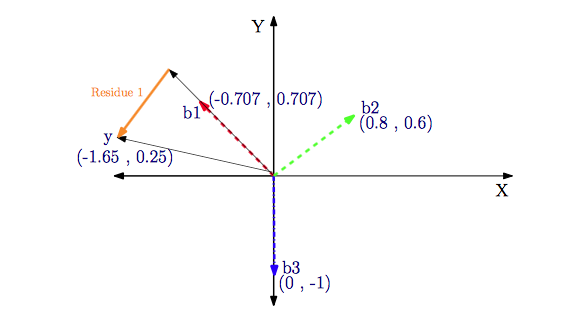•5星
135.91MB qq_34351067 2021-04-16 14:11:28
•5星
1KB qq_43045275 2021-05-20 17:09:55
•5星
17KB weixin_51307178 2021-02-27 21:58:52
•5星
3.95MB weixin_42696271 2021-09-10 15:15:58
•5星
251KB weixin_42696333 2021-09-10 21:01:43
•5星
30KB weixin_42325129 2021-06-14 23:11:58
•5星
7KB weixin_40663377 2021-09-04 17:31:39
•5星
40KB weixin_46567845 2021-08-06 21:39:04
•5星
1KB VampireCaptain 2021-04-23 10:07:12
•5星
5KB weixin_42696333 2021-09-10 15:53:15
• 955B qq_36787632 2017-03-22 21:31:39
• 基追踪匹配追踪MATLAB算法 MATLAB

2KB weixin_43638058 2020-11-29 17:27:26
• matlab正交匹配追踪算法 matlab

1KB dachengsoft01 2018-09-11 09:59:06
•5星
2KB weixin_42696333 2021-09-10 17:38:28
•4星
3KB weixin_40661440 2019-08-10 16:57:59
• 61KB qq_36596540 2020-05-31 16:23:23
• 正交匹配追踪算法（OMP）：正交匹配追踪算法（OMP）是一种贪婪的压缩感知恢复算法。-matlab开发 matlab

1KB weixin_38663973 2021-05-30 07:08:36
• 正交匹配追踪(OMP)的matlab程序 正交匹配追踪

2KB zhz18333601866zhzz 2019-01-06 09:29:12
• 匹配追踪MP、正交匹配追踪算法OMP，稀疏表示里的基本算法 matlab

228KB qq_43177596 2018-09-12 09:25:44
• 自适应匹配追踪matlab程序 匹配追踪

8KB qq_25156301 2017-12-13 09:22:19
• 5KB qq_42453715 2021-05-24 12:30:57
• 2KB leavemyleave 2021-10-10 21:47:39
• 2KB leavemyleave 2021-10-10 20:11:36
• 匹配追踪算法 python matlab 矩阵

匹配追踪算法是正交匹配追踪算法的基础，下面是matlab代码 function [x_rec] = MatchingPursuit( y, K, Theta) %% 匹配追踪算法 %% Vsesion:1.0 Written by zhenhuaLiu@ 2021.11.16 HIT ATCI %% 输入参数： %...

匹配追踪算法是正交匹配追踪算法的基础，下面是matlab代码

function [x_rec] = MatchingPursuit( y, K, Theta)
%% 匹配追踪算法
%% Vsesion:1.0 Written by zhenhuaLiu@ 2021.11.16 HIT ATCI
%% 输入参数：
%Theta:感知矩阵
%y: 压缩采样数据
%K: 稀疏度
%% 输出参数：
%x_rex: 重构的x向量
A = Theta;
[m, n] = size(Theta);
r = y;%初始化残差
x_rec = zeros(n, 1);%重构的稀疏向量初始化为0向量
A = Theta;
position = zeros(K, 1);%最大内积的索引值
max_inner = zeros(K, 1);%最大的内积值
for i = 1:K
% i = 2;
inner_product = r'*A;%计算残差与感知矩阵的内积；
[~, max_index] = max(abs(inner_product));% 找到内积的绝对值的最大值的下标
max_inner(i) = inner_product(max_index);%获得最大的内积值
r = r - max_inner(i)*A(:,max_index);
A(:, max_index) = zeros(m,1);%将支撑集索引对用的感知矩阵的该列全部置零
position(i) = max_index;
end
x_rec(position) = max_inner;  %重构的稀疏向量除了索引值位置之外其他位置都为0

展开全文wzz110011 2021-11-17 17:00:45
•4星
3KB hq98898 2017-10-31 20:33:50
• 实现正则化正交匹配追踪的算法代码，也就是ROMP实现的代码 matlab

1KB qq_43130544 2018-09-12 09:35:18
• 压缩感知的正交匹配追踪算法——matlab程序 matlab

1KB qq_43177596 2018-09-12 09:31:20
• MATLAB匹配追踪 MATLAB

记录：匹配追踪，以便回顾。 主要参阅以下网址:: https://chunqiu.blog.ustc.edu.cn/?p=634 https://chunqiu.blog.ustc.edu.cn/?p=646 http://blog.csdn.net/jbb0523/article/details/45099655 大致内容： 1...

记录：匹配追踪，以便回顾。

主要参阅以下网址::

https://chunqiu.blog.ustc.edu.cn/?p=634

https://chunqiu.blog.ustc.edu.cn/?p=646

http://blog.csdn.net/jbb0523/article/details/45099655

大致内容：

1.字典：为什么是完全的、冗余的

2.非线性表示

3.从字典到匹配追踪

4.怎样创建字典，以及创建什么样的字典，对信号进行匹配追踪恢复。

展开全文meteorhyl 2015-12-30 10:51:52
•5星
1019B xidianliutingting 2011-08-11 16:09:27
• Matlab从R2012a开始添加了匹配追踪(MatchingPursuit)函数，这个算法在稀疏表达领域是一个很常用的算法，这里对matlab函数做简答介绍，内容主要来自于matlab的doc帮助。Matlab对稀疏表达的说明简洁明了，翻译如下。1....

Matlab从R2012a开始添加了匹配追踪(Matching Pursuit)函数，这个算法在稀疏表达领域是一个很常用的算法，这里对matlab函数做简答介绍，内容主要来自于matlab的doc帮助。Matlab对稀疏表达的说明简洁明了，翻译如下。

1.冗余字典与稀疏性

用一组特定基表达一个信号其实就是找到相应的一组展开系数。虽然把信号用一组基表达，特别是一组正交基，有很多优点，但也存在缺点。

一组基表达信号的能力取决于信号的特性是否与基向量的特性相吻合。例如，光滑连续信号可以被傅里叶基稀疏的表达，但脉冲信号就不行。再如，带有孤立不连续点的平滑信号可以被小波基稀疏表达，但小波基在表达傅里叶频谱中有窄带高频支撑的信号时却是无效的。

现实世界中的信号经常包含有用单一基所不能表达的特征。对于这些信号，你或许希望可以选择来自不同基的向量(如用小波基和傅里叶基来联合表达一个信号)。因为你想保证你可以表达一个信号空间的所有信号向量，所以由所有可选向量组成的字典应该能够张成这个信号空间。然而由于这组字典中的向量来自不同的基，它们可能不是线性独立的。

由于这组字典中的向量不是线性独立的，会造成用这组字典做信号表达时系数不唯一。然而如果创建一组冗余字典，你就可以把你的信号展开在一组可以适应各种时频或时间-尺度特性的向量上。你 可以自由的创建包含多个基的字典。例如，你可以构造一组表达平方可积空间的基，这组基包含小波包基和局部余弦基。A wavelet packet basis is well adapted to signals with different behavior in different frequency intervals. A local cosine basis is well adapted to signals with different behavior in different time intervals. 这样构造的字典可以极大地增加你稀疏表达各种特性信号的能力。

2.字典非线性近似

定义表达你的信号空间的归一化基本模块作为字典。这些归一化向量叫做原子。如果字典的原子张成了整个信号空间，那么字典就是完全的。如果有原子之间线性相关，那么字典就是冗余的。在大多数匹配追踪的应用中，字典都是完全且冗余的。

假设{φk}表示字典的原子。假设字典是完全且冗余的。使用字典的线性组合表达信号将是不唯一的。一个重要的问题是是否存在一种最好的表达方式，一种直观的最好方式是选择φk使得近似信号和原始信号有最大的内积，如最好的φk满足即对于正交原子，为投影到由φk张成的子空间上的幅值。

匹配追踪的中心问题是你如何选择信号在字典中最优的M个展开项。

3.Matching Pursuit Algorithms匹配追踪算法

3.1 Basic Matching Pursuit(BMP)原始匹配追踪

令Φ(NxM,M>N)表示一个字典。如果这个完全、冗余的字典形成了信号空间的一个框架，那么可以使用框架算子得到使L2范数最小的一组展开系数，即然而，这个系数向量没有保留稀疏性。如果原始信号在字典空间中是稀疏的，那么使用标准框架算子得到的展开系数无法反映这个稀疏性。而原始信号的稀疏性是最想保留的特性。

对此，匹配追踪(Matching pursuit)可以直接得到信号稀疏性的表达。它本质是一种贪婪算法，通过计算信号在完全、冗余字典上的最佳非线性估计来实现。在计算过程中，一个稀疏近似序列被构建出来。令Φ={φk}表示一个原子归一化的字典，f表示信号。

1、首先定义R0f = f；

2、匹配追踪的第一步是从字典中找到与R0f的内积绝对值最大的原子，表示为φp；

3、通过从R0f减去其在φp所张成空间上的正交投影得到残差R1f4、对残差迭代执行2、3步5、直到达到某个指定的停止准则后停止算法。

在非正交匹配追踪(BMP)中，字典原子不是相互正交的向量。因此上面减去投影计算残差的过程中会再次引入与前面使用的原子不正交的成分。

为了更形象的看出这点，考虑下面的例子(这是一个类比，不代表匹配追踪过程)。考虑欧几里得二维空间的归一化框架字典假设有如下信号这个例子如图所示，字典原子以红色表示，信号向量蓝色表示在matlab中如下创建此字典和信号

dictionary = [1 0; 1/2 sqrt(3)/2; -1/sqrt(2) -1/sqrt(2)]';

x = [1 1/2]';

计算信号和字典原子的内积

scalarproducts = dictionary'*x;

内积绝对值最大的是原子[-1/sqrt(2); -1/sqrt(2)]。这很明显，因为两者之间的夹角约为π。从信号中减去信号在此原子上的正交投影得到残差，然后计算残差与其余原子的内积(因为残差是与此原子正交的，所以不用再计算与其的内积，此内积即是0)。

residual = x-scalarproducts(3)*dictionary(:,3);

scalarproducts = dictionary(:,1:2)'*residual;

这次内积绝对值最大的是[1;0]。所以迭代两次即得到了两个最佳原子[-1/sqrt(2);-1/sqrt(2)]和[1;0]。如果继续对残差迭代，你会发现输出不再与刚才找到的第一个原子正交。这意味着第一个原子有可能被再次选择到。这个事实及与收敛相关的问题在正交匹配追踪中得以解决。

3.2 正交匹配追踪Orthogonal Matching Pursuit

在正交匹配追踪OMP中，残差是总与已经选择过的原子正交的。这意味着一个原子不会被选择两次，结果会在有限的几步收敛。概念上讲，你可以通过施密特方法创建一个正交原子组来实现OMP。对于一组正交字典，你总会找到有限几个正交方向。OMP的算法如下

1、用f表示你的信号，初始化残差R0f=f；

2、选择与R0f内积绝对值最大的原子，表示为φp；

3、将选择的原子形成矩阵Φ的列，定义Φ列空间的正交投影算子为4、将此正交投影算子应用到残差上；

5、更新残差其中I为单位阵。OMP可以保证已经选择过的原子不会再被选择。

3.3 弱正交匹配追踪

如果放松选择原子的准则，将会带来计算量的有效降低这就是所谓的弱匹配追踪。

展开全文weixin_42300162 2021-04-20 00:42:27
• 匹配追踪算法（MP）简介 稀疏表征 匹配追踪

图像的稀疏表征 分割原始图像为若干个 ...匹配追踪算法可以直接得到信号稀疏性的表达. 以贪婪迭代的方法选择 D \mathrm{D} 的列，使得在每次迭代的过程中所选择的列与当前冗余向量最大程度的相关.

图像的稀疏表征

分割原始图像为若干个

n×n
的块. 这些图像块就是样本集合中的单个样本 y=Rn $y = \mathbb{R}^n$. 在固定的字典上稀疏分解 y $y$后，得到一个稀疏向量. 将所有的样本进行表征一户，可得原始图像的稀疏矩阵. 重建样本y=Rn$y = \mathbb{R}^n$时，通过原子集合即字典 D={di}ki=1Rn×m(n<m) $\mathrm{D} = \{d_i\}^k_{i=1} \in \mathbb{R}^{n \times m} (n < m)$中少量元素进行线性组合即可：

y=Dx

其中， x={x1,x2,,xm}Rm $x = \{x_1, x_2, \cdots, x_m\} \in \mathbb{R}^m$ y $y$D$\mathrm{D}$上的分解系数，也称为稀疏系数.

字典矩阵中的各个列向量被称为原子（Atom）. 当字典矩阵中的行数小于甚至远小于列数时，即 mn $m \leqslant n​$，字典 D $\mathrm{D}​$是冗余的。所谓完备字典是指原子可以张成 n $n​$纬欧式空间 y=Rn $y = \mathbb{R}^n​$. 如果在某一样本在一过完备字典上稀疏分解所得的稀疏矩阵含有大量的零元素，那么该样本就可以被稀疏表征，即具有稀疏性。一般用 l0 $l_0​$范数作为稀疏度量函数，图像的稀疏表征数学模型如下：

minx||x||0,s.t.y=Dx

稀疏表征不仅具有过完备性，还应该具有稀疏性。对于一个过完备字典 D $\mathrm{D}$，为了可以分解出更合适且稀疏的稀疏表征，应当含有更多的原子。

在稀疏表征理论方面的研究主要可分为两个方面:字典的构建和稀疏编码.

稀疏编码的目标就是在满足一定的稀疏条件下，通过优化目标函数，获取信号的稀疏系数. 经典的算法有匹配追踪(Matching Pursuit，MP)、正交匹配追踪(Orthogonal Matching Pursuit，OMP)、基追踪(Basis Pursuit，BP)算法等.

MP算法是稀疏表征中用于稀疏求解的最基本方法之一. 我在学习过程中参考网上一些资料，觉得大部分写得比较理论化，看起来稍微吃力一些. 阅读了Koredianto Usman的Introduction to Matching Pursuit(MP)一文，我觉得这篇文章写得很不错，从实例出发，很好接. 这篇博文是我对该文章翻译的基础上而写的.

注：
1. 原文中有一些小错误，我在译文中进行了修改. 有对照原文阅读的同学，若发现有不一致，请不要奇怪.
2. 所有计算结果都保留两位小数.

问题提出

考虑下面一个简单例子：

给定稀疏信号

x=1.210

字典矩阵A为：

A=(0.7070.7070.80.601)

（注：原文中称 A $\mathrm{A}$为measurement matrix）

所以， y=Ax=(1.650.25) $y=\mathrm{A} \cdot x=\begin{pmatrix}1.65 \\ -0.25\end{pmatrix}$

现在，给定 y=(1.650.25) $y=\begin{pmatrix}1.65 \\ -0.25\end{pmatrix}$ A=(0.7070.7070.80.601) $\mathrm{A}=\begin{pmatrix}-0.707 & 0.8 & 0 \\ 0.707 & 0.6 & -1\end{pmatrix}$,

如何求得 x $x$呢？

匹配追踪

在上面的列子中A$\rm{A}$中的列向量称之为Basis（基）或者Atoms（原子）. 所以，我们有如下原子：

b1=(0.7070.707)b2=(0.80.6)b3=(01)

因为 A=[b1b2b3] $\rm{A} = \begin{bmatrix}b_1 & b_2 & b_3\end{bmatrix}$，如果我们令 x=[abc] $x = \begin{bmatrix}a & b & c\end{bmatrix}$，则 Ax=ab1+bb2+cb3 $\mathrm{A}\cdot x = a\cdot b_1 + b\cdot b_2 + c\cdot b_3$.

Ax $\mathrm{A}\cdot x$是原子 b1 $b_1$ b2 $b_2$ b3 $b_3$的线性组合

Ax=(0.7070.7070.80.601)1.210=1.2(0.7070.707)+1(0.80.6)+0(01)=y=(1.650.25)

从上面的方程可以看出， b1 $b_1$ y $y$值的贡献最大，然后是b2$b_2$，最后是 b3 $b_3$. 匹配追踪算法刚好逆方向进行计算：我们首先从 b1 $b_1$ b2 $b_2$ b3 $b_3$中选出对 y $y$值贡献最大的，然后从差值（residual）中选出贡献次大的，以此类推.

而贡献值的计算通过内积（点积）进行计算，MP算法步骤如下：

1. 选择对y$y$值贡献最大的原子 pi=maxj<bj,y> $p_i=\max_j$

• 计算差值 ri=ri1pi<ri1,pi> $r_i = r_{i-1} - p_i \cdot $ （注：该公式在原文中稍微有点问题，这里做了修正. 对于 r0=y $r_0=y$
• 选择剩余原子中与 ri $r_i$内积最大的
• 重复步骤2和3，直到差值小于给定的阈值（稀疏度）
• 下面进行实例计算：

首先，分别计算 y $y$b1$b_1$ b2 $b_2$ b3 $b_3$的内积：

<y,b1>=1.34,<y,b2>=1.17,<y,b3>=0.25

取绝对值以后，我们可以发现 b1 $b_1$ y $y$得到最大的内积值. 然后，在第一步中我们选择b1$b_1$. 接下来计算差值：

r1=yb1<y,bi>=(1.650.25)(1.34)(0.7070.707)=(0.700.70) $r_1 = y - b_1 \cdot = \begin{pmatrix}1.65 \\ -0.25\end{pmatrix} - (-1.34) \cdot \begin{pmatrix}-0.707 \\ 0.707\end{pmatrix} = \begin{pmatrix}0.70 \\ 0.70\end{pmatrix}$

接来下，计算差值和 b2 $b_2$ b3 $b_3$的内积：

<r1,b2>=0.98<r1,b3>=0.70
<script type="math/tex; mode=display" id="MathJax-Element-58"> =0.98 \qquad =-0.70</script>

取绝对值以后， b2 $b_2$ y $y$值的贡献最大。

接下来，计算差值r2=r1b2<r1,b2>=(0.70.7)(0.80.6)0.98=(0.080.11)$r_2 = r_1 - b_2 \cdot = \begin{pmatrix}0.7 \\ 0.7\end{pmatrix} - \begin{pmatrix}0.8 \\ 0.6\end{pmatrix} \cdot 0.98 = \begin{pmatrix}-0.08 \\ 0.11\end{pmatrix}$

最后，计算 r2 $r_2$ b3 $b_3$的内积： <r2,b3>=0.11 <script type="math/tex" id="MathJax-Element-64"> =-0.11</script>

所以，最后的三个稀疏稀疏是 1.340.980.11 $\begin{pmatrix}-1.34 \\ 0.98 \\ -0.11\end{pmatrix}$

这和准确的系数 1.210 $\begin{pmatrix}-1.2 \\ 1 \\ 0\end{pmatrix}$很接近

反酸回去，和给定的 y $y$也很接近.

Ax=(0.7070.7070.80.601)1.340.980.11=(1.730.25)

MP算法实质

从下面的图，我们可以很清楚地看到MP算法的实质：就是利用原子向量的线性运算去逐渐去逼近信号向量，经过不停地迭代，最后达到给定的稀疏度.

匹配追踪算法可以直接得到信号稀疏性的表达. 以贪婪迭代的方法选择 D $\mathrm{D}$的列，使得在每次迭代的过程中所选择的列与当前冗余向量最大程度的相关.展开全文T_27080901 2017-10-11 23:17:17
• 块正交匹配追踪：使用 BOMP 算法的压缩系统识别。-matlab开发 matlab

8KB weixin_38627234 2021-05-30 11:44:35
• 压缩感知——浅析匹配追踪算法（Matching Pursuit，MP）与正交匹配追踪算法（Orthogonal Matching Pursuit... 算法

ikhui7 2019-12-23 22:07:33
• 一分钟了解”匹配追踪算法（Matching Pursuit，MP） 和 正交匹配追踪算法（Orthogonal Matching Pursuit，... 匹配追踪算法 正交匹配追踪算法 OMP

yes1989yes 2018-08-18 14:04:59
• matchPurs.m:经典的匹配追踪算法-matlab开发 matlab

2KB weixin_38570854 2021-06-01 14:54:42
•5星
4KB snoopylm 2011-11-24 15:04:29...

matlab匹配追踪算法matlab 订阅